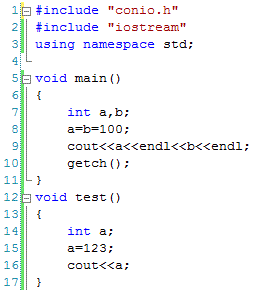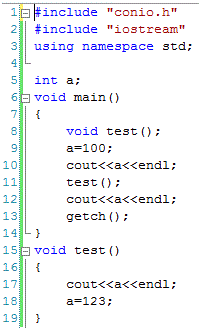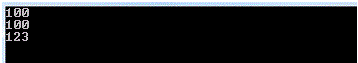# C++ Variables

## C++ Variables, Its types and Scope in C++

Almost all C++ programs need to get input from user in order to produce required information after processing. When we take inputs from user then these input values should be stored somewhere in computer RAM so that they are available for processing. For this purpose we define variables. For every variable a space is reserved in computer RAM where we can store and read the data.  Variables are not only used to store the input values but can be used to store any data required to produce the required results.

Variables vs. Constants

The word ‘variable’ is derived from ‘vary’ which means to change. So variables are changeable values. Some values used in computer programs are fixed or constant. We cannot change their values or meanings. They are called constant (const in C++).  For instance, the value of PI is 3.14 and this is fixed as we cannot change the value of PI. On the other hand, speed of a car is a variable quantity as the speed of car changes according to the road conditions. Our age is also a variable quantity as our age is growing after every second.

Declaration of Variables

If we want to use a variable in C++ program, then it must be declared before using. While declaring a variable we need to give a meaningful name to it along with the data type. The data type shows that what kind of data can be stored in that variable. A number of data types are available in C++ as discussed in the last post, but the commonly used data types are int, float, double, char and bool.

Rules to Define a Variable Name

While declaring a variable, we need to follow the following rules.

Every variable must be given a proper name. A variable name may consist of the following characters:

• Alphabetic characters (A to Z, a to z)
• Numeric characters (0 to 9)
• Underscore (_)

Special characters (except underscore) are not allowed to be used in variable names.
Digits can’t be used as a first character of a variable name.
Keywords (already defined in C++) are not allowed to be defined as variable names.
Variable names are case sensitive as other statements of C++.
Spaces are not allowed in variable names.

Examples of Some Valid Variable Names

int marks;
int my_marks;
double average;
float _speed;
float _abc_;
int a_b_c;
int _a_b_c_;
int marks1;
char c123;
int abc123xyz;

Examples of Some Invalid Variable Names

int my marks; (spaces are not allowed)
int my.marks; (special characters including dot is not allowed)
float 123speed; (digits may be at the end or middle but not at start)
float int; (keyword cannot be used as a variable name)

Assigning Values to Variables

We can assign values to a variable in a number of ways. Some the them are as below.

 int marks; marks = 76; Variable marks is first declared and then a value is assigned to it. As the data type is int so the value of marks must also be integer otherwise we will get an error int a=100; This is called variable initialization. The required value can be assigned at the time of variable declaration char ch=’c’; Character values are enclosed in single quotes int a, b; a=100; b=100; We can define more than one variable using common data type in same statement. The vales can be assigned as shown. int a, b; a=b=100; If both variables have same value then same statement can be used as shown in the example. int a; cin>>a; cin is used to get the value from user. When the user enters a value then it will be stored in the specified variable. int a=1, b=2; int c=a+b; Result of an expression can be stored in a variable

Scope of Variables

When we declare a variable then it can only be used in the scope boundary assigned to it. In a single C++ program we can define one or more functions. We will discuss about functions later in detail but at the moment you should know that function is a piece of code written to perform a specific task.

If we have more than one tasks to be performed in the same program then we can write more than one function for each task. To perform these tasks, we need to define variables in the functions along with some other code. If we define a variable in a specific function then that variable can be used in that specific function only.

The scope of a variable is the function where the variable is declared. Sometimes, a variable is required to be used in all the functions of a program. For this purpose the function is not defined in a function but outside the function. If a variable is defined outside a function then it is not the property of any function and thus can be used in all the remaining functions. The scope of that variable is not a specific function but the whole program where the variable is declared.

Local Variables

If a variable is defined in a function then the variable is called local variable for that function. Local variables can only be used in the function where declared. For example in the following code there are two functions  main() and test(). The variables declared in main are local for main () and can only be used in this function.

Similarly the variable declared in test () function is local for this function and can be used in this function only. If the same variable name is used in more than one functions then this doesn’t mean that the same variable is declared, rather each variable has different meaning to the compiler and have their own separate memories in RAM.

In the following example variable ‘a’ is declared in both the functions but the variable declared in main() is different than the variable ‘a’ declared in test() function.Global Variables

If a variable is declared outside the function then the variable is called global variable and can be used in more than one functions of the program. If one function stores a value in the global variable then the same value will be retrieved by other functions of the program. Any function of the same program can change or retrieve the value of the global variable as shown in the code below.

In the following code variable ‘a’ is declared outside of any function and above main(). It becomes global and can be used in all the functions of the program. We can also see that the value of this global variable can be retrieved or changed in both the functions.The output of the program is as belowFinal Variables

Sometimes we want that the value of a variable once assigned should not be changed later. These kind of variables are called final or constant variables. The value of these variables are initialized at the time of declaration and cannot be changed later. Both local and global variables can be declared as final variables. Final variables are declared as below:

const int a=100;

‘const’ keyword is used to declare final variables and the variable must be given a value at the time of declaration which will remain constant throughout the program.